• 直接法求解循环谱密度，画出3D图，并与参考文献中的代码进行比较。
• 分析了循环自相关函数和循环谱密度函数的解调性能、对噪声的免疫性能及其运算量大的局限性,兼顾故障特征提取的准确性和计算量,提出了峰值频率切片集合分析法:取循环自相关切片图上的各峰值频率作为循环频率分别做...
• 源网址：http://blog.sciencenet.cn/blog-825323-636888.html 功率谱密度函数估计，在随机信号处理中具有极其重要的意义。不管是为了目前增加对信号基本属性的了解，还是为了以后对信号作进一步分析处理，现在对...
源网址：http://blog.sciencenet.cn/blog-825323-636888.html  功率谱密度函数估计，在随机信号处理中具有极其重要的意义。不管是为了目前增加对信号基本属性的了解，还是为了以后对信号作进一步分析处理，现在对敝人各生理信号作一下功率谱估计，都是很有必要的。    MATLAB信号处理工具sptool中，有8种已经很成熟的功率谱估计方法(FFT法，Welch法，Covariance法，Mod.Covariance法，MTM法，MUSIC法，Burg法，Yule AR法)可供调用，非常方便。    将体温信号序列TW461512_0导入sptool，并Create（加载）于spectra栏，即打开功率谱处理、观察窗口Spectrum Viewer。    （1）、先看看快速傅立叶变换FFT方法,也就是所谓的经典周期图法吧。取FFT执行点数Nfft=2^16=65536。在2的n次幂中，它比TW461512_0的长度55380长，且最接近55380。采样频率Fs取Fs=Nfft=65536（次/单位采样时间，我亦称之为“Hz”，将“1次/单位采样时间”的采样频率提高Nfft倍，是为了使频率轴都用正整数表示。图6-1 TW461512_0快速傅立叶FFT法估计的功率谱图     此图中左标尺所标示的谱线位置，为频率f=5461Hz处。其波形周期T=Fs/f=65536Hz/5461Hz=12.0007单位采样时间，即1天。其小数点后面的误差为数字化误差。此谱线右边的谱线为其倍频谱线。图6-2 FFT法功率谱最低频处。频率轴放大128倍，幅值轴放大4倍。     波峰狭窄尖锐，幅值相近，噪声谱与信号谱混杂在一起，很难分辨样本信号谱峰。图6-3 FFT法功率谱最高频处。放大倍数同前。    谱峰形状与最低频处一样。     （2）、来看看使用Welch（经典的韦尔奇）方法估计功率谱。此方法需要选择的参数比较多。除了FFT执行点数Nfft外，还需要确定窗函数Window及其长度Nwind、分段重叠点数Overlap。对于没有足够的先验知识的人来说，要一次选对各个参数是很难的。先比较随意地选定一组参数：Window=hanning，Nwind=4096，Overlap=1024，作一功率谱图如下：图6-4 TW461512_0经典welch法估计的功率谱图图6-5 图6-4最低频处。横轴放大16倍，纵轴放大1.2倍。图6-6 图6-4最高频处。横轴放大16倍，纵轴无放大。    直觉上感到此谱图各波形太过平缓，波峰幅值相近，难以确定信号波峰及其频率点。现在以窗函数宽度及其重叠的比例为变量，作搜索式计算。编程如下： %welch法搜索参数L=length(TW461512_0);%=55380;m0=512;%窗函数最小宽度c=floor(L/(2*m0));%信号序列最大分段数for i=1:4M=zeros(300,c);for j=1:cm=j*m0;%窗宽按每次增加一个最小窗宽数m0搜索n=m*(i-1)/4;%数据分段重叠比例Pxx=PWELCH(TW461512_0,hanning(m),n,65536,65536);M(1:149,j)=Pxx(1:149);%数据太长,无法全部清晰作图显示,只能%取最低频与最高频段各149点M(150:151,j)=ones(2,1);%人工隔板M(152:300,j)=Pxx(length(Pxx)-148:length(Pxx));endM=M';%横坐标表示频率,纵坐标表示窗宽figure(i)surf(log10(M))end     运行,得:图6-7 TW461512_0的Welch法估计功率谱。分段无重叠图6-8 TW461512_0的Welch法估计功率谱。分段重叠比例为1/4图6-9 TW461512_0的Welch法估计功率谱。分段重叠比例为2/4图6-10 TW461512_0的Welch法估计功率谱。分段重叠比例为3/4     由于数据太长，无法在一张图片中显示整个结果，只能分别取最低频最高频段各149Hz合在一起显示。中间的“隔板”是另加的。    上面四张图片，分别是信号序列分段无重叠、重叠1/4、2/4、3/4时的谱图。其纵坐标表示窗函数的宽度（以512点为一个单位）。我认为谱估计效果最好的应该是最后一张图，即信号序列分段重叠3/4的时候。因为它在整个纵轴方向上，随着窗宽增加，波峰略为变高变窄，而位置（频率点）都很少改变，而不像前面三张图，各波形波峰高度及其频率点都随着窗函数宽度的改变而有所改变，尤其是窗函数宽度比较小的时候。    我认为一种好的估计方法，当需要选择的参数落在一个大范围后，其估计结果应该对参数的变化是“不敏感”的。原因很简单：真理只有一个。如果反之，估计结果对参数的变化是“敏感”的，参数稍微一变化，估计结果就跟着很快变化，那么我们最终到底要选哪一个参数？如果随便选一个，那岂不就象买彩票一样？科学要追求确定性的结果，而不是中彩。而这里的参数必定是由人来选定的。如果参数可以由一套算法来计算、确定，那就不用设参数，而设置一个函数好了。    根据上面的分析，本信号序列采用Welch方法估计功率谱时，窗宽重叠率须取1/2以上。至于窗宽，取一个中间偏大的值如512×（35～54）都可（第一行的窗宽为512点，最后一行窗宽为512×54＝27648点）。谱图图片暂就不贴了，等到在同一窗口比较各方法谱估计曲线时一并贴出。     （3）Covariance(协方差）法。此法需选择参数，除了快速傅立叶变换执行点数Nfft，就是阶数Order了。取Nfft＝65536，任取几个阶数Order运行，发现此法非常耗用计算机资源。当Order>500时即Out of memory（超出内存）了。    我的内存为2×1G。要增加内存，牵涉到整机升级的问题，不是换两根内存条就可以了的。据说可以设置虚拟内存，但我设置之后，也没见运算能力的明显提高。不知是何缘故。    自己编程，看看Order<=500时的谱估计情况： %Covariance法搜索阶数的计算M=zeros(300,10);for i=1:10order=50*i;Pxx=pcov(TW461512_0,order,65536,65536);M(:,i)=Pxx(1:300);endM=M';surf(log10(M))     运行，得：图6-11 Covariance法搜索阶数的计算    什么也没有。所以在个人电脑上是无法使用Covariance(协方差）法的。     （4）、Mod.Covariance（改进的协方差）法。在Spectrum Viewer窗口中略一运行，便知此法比Covariance法更耗电脑资源。pass。     （5）、MTM（Multitaper多窗口）法。此方法需要选择的参数有时间-带宽乘积nw、fft执行点数Nfft、采样频率Fs、权重算法Weights。    取Nfft=Fs=65536，Weights='adapt'（Thomson的非线性自适应组合算法），各取nw=2、3、4，得到3条谱估计曲线。使3条曲线在同一窗口显示，见图6-12。图6-12 时间-带宽乘积nw不同，其余参数相同时，TW461512_0的功率谱估计曲线。其中绿线nw=2,红线nw=3,蓝线nw=4。图6-13 图6-12最低频段处。横轴放大128倍，纵轴放大（为原来）2倍。图6-14 图6-12最高频段处。横轴放大128倍，纵轴放大（为原来）2倍。     图中3条曲线在大的走势上虽然保持了一致，但在更细节的形状上差异也不小，而且很多波峰形状也不明显，幅值接近，使人很难判断真实的谱线。    此方法中，2*nw-1表示采用的窗口数。nw的典型取值有2，5/2，3，7/2，4。但我发现在上述取值范围内，谱估计函数Pxx=pmtm(x,nw,Nfft,Fs,Weights)对所有实数nw都是有意义的。仿照我前面搜索welch法参数的方法，作搜索nw的计算。程序如下： %搜索MTM谱估计法中时间-带宽乘积参数nwNfft=65536;fft执行点数Fs=65536;%采样频率M=zeros(300,30);for i=16:45nw=0.1*i;%时间-带宽乘积Pxx=pmtm(TW461512_0,nw,Nfft,Fs,'adapt');M(1:149,i)=Pxx(1:149);%最低频段149HZM(150:151,i)=ones(2,1);%人造隔板M(152:300,i)=Pxx(length(Pxx)-148:length(Pxx));%最高频段149HZendM=M';surf(log10(M))    运行，得：图6-15 TW461512_0的pmtm法估计功率谱。权重算法为adapt     觉得还是很难确定nw到底取多大为好。改程序中权重算法因子为unity（一致权重的线性组合算法）、eigen（特征值权重的线性组合算法），运行，得：图6-16 TW461512_0的pmtm法估计功率谱。权重算法为unity。图6-17 TW461512_0的pmtm法估计功率谱。权重算法为eigen。     发现上面三张图中，曲面形状非常一致。注：上面三张图及图6-7～图6-10的纵坐标应乘10才是分贝值，因为程序中“surf(log10(M))”应为“surf(10*log10(M))”。懒得再截图了，说明一下。    回到Spectrum Viewer窗口，取定nw=3，Nfft也不变，分别取Weights='adapt'、'unity'、'eigen'，得到3条曲线。令其在同一窗口显示，如下图：图6-18 Weights不同，其余参数相同时，TW461512_0的功率谱估计曲线。最低频段处，横轴放大128倍，纵轴放大为2倍。其中绿线Weights='eigen'，红线Weights='unity'，蓝线Weights='adapt'     可以看出，最低频段处，3条曲线基本上重合了，后面的绿线、蓝线基本上都被前面的红线挡住。图6-19 TW461512_0的功率谱估计曲线。最高频段处。其余情况同图6-18    最高频段处，绿线与红线曲线基本上重合了，蓝线在某些部位不与红线、绿线重合。    所以，MTM法中参数Weights的选择是不敏感的，选哪一个都差不多。   （6）、MUSIC（多信号分类）法。该法需要选择的参数比较多，有信号子空间数Signal Dim.、阈值、fft长度Nfft、窗函数、窗函数宽度Nwind、窗函数重叠点数Overlap。随选几组参数,在Spectrum Viewer窗口试运行,即知此方法非常占用电脑资源。信号子空间Signal Dim.参数达到4000、加窗宽度Nwind达到7680（=512*15）时便“Out of memory”了。    现在我觉得，Matlab中Sptool的Spectrum Viewer窗口功率谱估计，最适合的是那些做固定项目工程应用的人。他们对谱估计相关的参数选择都已经很熟悉，只是由于样本数据的变化，而在此窗口查看一下谱估计的结果。对于做科研工作的人来说，大多数是第一次接触到新项目，谱估计参数选择不熟悉。此窗口只能作辅助性的研究工具。    准备看看在Signal Dim.<=4000、Nwind<=7680时的功率谱估计情况。先固定窗函数及其宽度、窗函数重叠点数，对Signal Dim.作搜索计算。程序在傍晚时开始运行，结果运行到第二天临晨还没有结束，只好强行中断。虽然感觉再要不了多久就会结束，但也不是十分有把握。如果还需要运行几十、几百……个小时，那不麻烦了。第二天先试循环少量几次，对整个程序的运行时间有一个大概的评估后才开始正式的运行。所以对有循环语句的程序，这个工作是少不了的，除非非常有经验。    将原程序略作改动，分段运行： pack%整理工作空间Nfft=65536;fft执行点数Fs=65536;%采样频率d=100;%每段程序循环搜索次数k=20;%信号子空间数每次增加数Dim0=2000;M_2000_20_4000=zeros(300,d);m=5120;%窗函数宽度n=m/2;%数据分段重叠比例for i=1:dDim=Dim0+i*k;Pxx=pmusic(TW461512_0,Dim,Nfft,Fs,hanning(m),n);M_2000_20_4000(1:150,i)=Pxx(1:150);M_2000_20_4000(151:300,i)=Pxx(length(Pxx)-149:length(Pxx));endM_2000_20_4000=M_2000_20_4000';     运行完毕后，将其中d=100; k=20;Dim0=2000;分别改成d=100、100、75;k=10、7、4;Dim0=1000、300、0;M_2000_20_4000改成M_1000_10_2000、M_300_7_1000、M_0_4_300，分4段运行。4段程序大约运行了15、6个小时。    最后：M=[M_0_4_300;M_300_7_1000;M_1000_10_2000;M_2000_20_4000];surf(10*log10(M))    运行，得：图6-20 TW461512 _ 0的pmusic法估计功率谱，对信号子空间Signal Dim.的搜索。hanning窗宽5120点，重叠2560点    可以看出，Signal Dim.太小的时候，基本上没有什么谱峰。即便在Signal Dim.最大值4000处，谱峰也很平缓。这是低频段的情况。在高频段则始终没有出现谱峰。    总而言之，本人现在的电脑配置是没法使用MUSIC方法估计本样本数据的功率谱的。最后再贴几张不同参数下的功率谱估计图吧：图6-21 Dim=4000，Nwind=15*512=7680图6-22 Dim=4000，Nwind＝7*512=3584图6-23 Dim=3200，Nwind=2*512=1024图6-24 Dim=1000，Nwind=2*512=1024      （7）、Burg法。前面6种估计方法都是属于非参数估计方法，Burg法及最后的Yule AR法属于参数估计方法。在Spectrum Viewer窗口中，Burg法需要选择的参数，除了fft长度Nfft，就只有阶数Order。试选择几个Order数运行，知道其可运行范围很大（Order=20000都可以）。反正我对于Order的具体选择也没有经验，那就编程作搜索式运算吧。 %用pburg法估计TW461512_0功率谱，搜索阶数参数OrderNfft=65536;M=zeros(Nfft/2+1,240);for i=1:240Order=50*i;Pxx=pburg(TW461512_0,Order,Nfft);M(:,i)=Pxx;endP_TW4615_b50_50_12000=M;%保存M(151:(Nfft/2+1)-150,:)=[];M=M';surf(10*log10(M))     运行，得：图6-25 用pburg法估计TW461512_0功率谱，搜索阶数参数Order     上图数据太密集，图形太暗。 M(1:2:240,:)=[];%删去一部分数据surf(10*log10(M))图6-25(1) 用pburg法估计TW461512_0功率谱，搜索阶数参数Order     可以看出，低频段，阶数达到8、9000以上，高频段，阶数达到5、6000以上后，谱峰形状与位置都很稳定。这也符合我在用welch方法估计功率谱时所说的“我认为一种好的估计方法，当需要选择的参数落在一个大范围后，其估计结果应该对参数的变化是“不敏感”的”的特点。    贴几张Spectrum Viewer窗口谱图：图6-26 用pburg法估计TW461512_0功率谱，阶数Order=10000图6-27 图6-26最低频段处，横轴放大128倍，纵轴放大为2倍图6-28 图6-26最高频段处，横轴放大128倍，纵轴放大为2倍     从波形上来看，总的感觉，参数估计法比非参数估计法效果就是要好得多。      （8）、Yule AR法。此法与Burg法极其相似，在Spectrum Viewer窗口中需选择的参数完全一样。搜索阶数Order的程序只需将其谱估计函数“pburg”换成“pyulear”就可以了。 %用pyulear法估计TW461512 _ 0功率谱，搜索阶数参数Order。Nfft=65536;M=zeros(Nfft/2+1,120);for i=1:120Order=100*i;Pxx=pyulear(TW461512_0,Order,Nfft);M(:,i)=Pxx;endP_TW4615_y100_100_12000=M;%保存M(151:(Nfft/2+1)-150,:)=[];M=M';surf(10*log10(M))     运行，得：图6-29 用pyulear法估计TW461512_0功率谱，搜索阶数参数Order     第一感觉，就是此图与图6-25也极其相似。再贴几张Spectrum Viewer窗口谱图：图6-30 用pyulear法估计TW461512_0功率谱，阶数Order=10000图6-31 图6-30最低频段处，横轴放大128倍，纵轴放大为2倍图6-32 图6-30最高频段处，横轴放大128倍，纵轴放大为2倍    感觉上面三图与图6-26、图6-27及图6-28也极其相似。将图6-27与图6-31、图6-28与图6-32中的曲线分别放在同一窗口中进行比较。见下二图：图6-33 最低频段处。蓝线是Burg法、红线是Yule AR法、绿线是Welch法图6-34 最高频段处。其余情况同上图     绿线Welch法谱估计参数是hanning窗，窗宽Nwind=25600,窗函数重叠点数Overlap=19200。    可以看到，蓝线与红线重叠程度非常高。绿线作为一种非参数估计方法，其曲线形状变化步调与蓝线、红线能够保持这么高，已经很不简单了。    上面就目前最常用的8种谱估计方法，对体温信号序列TW461512_0作了初步的功率谱估计。对这些谱估计的结果作进一步的分析处理，就放到以后的篇目中再做。 （本文首发于：http://blog.sina.com.cn/s/blog_6ad0d3de0100otj3.html首发时间：2011-01-21 11:11:50）  转载本文请联系原作者获取授权，同时请注明本文来自柏世平科学网博客。链接地址：http://blog.sciencenet.cn/blog-825323-636888.html
展开全文• 8 QPSK信号相关密度函数 8.1 实信号模型 QPSK实信号表达式可以写为 r(t)=yI(t)−yQ(t)+n(t)r(t) = {y_I}(t) - {y_Q}(t) + n(t)r(t)=yI​(t)−yQ​(t)+n(t) =c(t)pI(t)−s(t)pQ(t)+n(t)= c(t){p_I}(t) - s(t){p_Q}...
说明：接上一节循环自相关函数和谱相关密度（三）——实信号、复信号模型下的BPSK信号循环谱MATLAB仿真结果及代码
8 QPSK信号谱相关密度函数
8.1 实信号模型
QPSK实信号表达式可以写为
$r(t) = {y_I}(t) - {y_Q}(t) + n(t)$
$= c(t){p_I}(t) - s(t){p_Q}(t) + n(t)$
$= \sum\limits_{n = - \infty }^\infty {c(nT)q(t - nT - {t_0})} \cos (2\pi {f_0}t + \theta )$
$+ \sum\limits_{n = - \infty }^\infty {s(nT)q(t - nT - {t_0})} \sin (2\pi {f_0}t + \theta ){\text{ + }}n(t)$	(54)
其中，${t_0}$为起始时间，$T$为符号速率，$c(n)$和$s(n)$为基带符号序列，${f_0}$为载波频率，$\theta$为初始相位，$n(t)$为高斯白噪声，$q(t)$为矩形脉冲，且
$c(t) = \sum\limits_{n = - \infty }^\infty {a(nT)q(t - nT - {t_0})}$
$= q(t - {t_0}) \otimes \sum\limits_n {a(t)\delta (t - nT)}$
$= q(t - {t_0}) \otimes \hat a(t)$	(55)
$s(t) = \sum\limits_{n = - \infty }^\infty {b(nT)q(t - nT - {t_0})}$
$= q(t - {t_0}) \otimes \sum\limits_n {b(t)\delta (t - nT)}$
$= q(t - {t_0}) \otimes \hat b(t)$	(56)
${p_I}(t) = \cos (2\pi {f_0}t + \theta )$	(57)
${p_Q}(t) = \sin (2\pi {f_0}t + \theta )$	(58)
考虑${p_Q}(t)$的二次变换
${v_{Q\tau }}(t) = {p_Q}(t{\text{ + }}\tau /2)p_Q^*(t - \tau /2)$
$= \frac{1}{4}({e^{j2\pi {f_0}\tau }} + {e^{ - j2\pi {f_0}\tau }} - {e^{j(4\pi {f_0}t + 2\theta )}} - {e^{ - j(4\pi {f_0}t + 2\theta )}})$	(59)
其Fourier级数系数为
${\left\langle {{v_{Q\tau }}(t){e^{ - j2\pi \alpha t}}} \right\rangle _t} = \frac{1}{4}{e^{j2\pi {f_0}\tau }}{\left\langle {{e^{ - j2\pi \alpha t}}} \right\rangle _t} + \frac{1}{4}{e^{ - j2\pi {f_0}\tau }}{\left\langle {{e^{ - j2\pi \alpha t}}} \right\rangle _t}$
$- \frac{1}{4}{e^{ - j2\theta }}{\left\langle {{e^{ - j2\pi (\alpha + 2{f_0})t}}} \right\rangle _t} - \frac{1}{4}{e^{j2\theta }}{\left\langle {{e^{ - j2\pi (\alpha - 2{f_0})t}}} \right\rangle _t}$	(60)
则${p_Q}(t)$的循环自相关函数和谱相关密度函数为
$R_{p_{Q}}^{\alpha}(\tau)=\left\{\begin{array}{cc}-\frac{1}{4} e^{\pm j 2 \theta} & \alpha=\pm 2 f_{0} \\ \frac{1}{2} \cos \left(2 \pi f_{0} \tau\right) & \alpha=0 \\ 0 & \text { otherwise }\end{array}\right.$(61)
$S_{p_{Q}}^{\alpha}(f)=\left\{\begin{array}{cc}-\frac{1}{4} e^{\pm j 2 \theta} \delta(f) & \alpha=\pm 2 f_{0} \\ \frac{1}{4}\left[\delta\left(f+f_{0}\right)+\delta\left(f-f_{0}\right)\right] & \alpha=0 \\ 0 & \text { otherwise }\end{array}\right.$(62) 由(12)、(13)得${y_Q}(t)$的循环自相关函数和谱相关密度函数为 $R_{{y_Q}}^\alpha (\tau ) = \sum\limits_\beta {R_{{p_Q}}^\beta (\tau )R_s^{\alpha - \beta }(\tau )}$ $= - \frac{1}{4}{e^{j2\theta }}R_s^{\alpha - 2{f_0}}(\tau ) - \frac{1}{4}{e^{ - j2\theta }}R_s^{\alpha + 2{f_0}}(\tau ) + \frac{1}{2}\cos (2\pi {f_0}\tau )R_s^\alpha (\tau )$ (63) $S_{{y_Q}}^\alpha (f) = \sum\limits_\beta {S_{{p_Q}}^\beta (f) \otimes S_s^{\alpha - \beta }(f)}$ $= \frac{1}{4}\left[ {S_s^\alpha (f + {f_0}) + S_s^\alpha (f - {f_0}) - {e^{j2\theta }}S_s^{\alpha - 2{f_0}}(f) - {e^{ - j2\theta }}S_s^{\alpha + 2{f_0}}(f)} \right]$ (64) 由(35)可得${y_I}(t)$的谱相关密度函数为 $S_{{y_I}}^\alpha (f) = \sum\limits_\beta {S_{{p_I}}^\beta (f) \otimes S_c^{\alpha - \beta }(f)}$ $= \frac{1}{4}\left[ {S_c^\alpha (f + {f_0}) + S_c^\alpha (f - {f_0}) + {e^{j2\theta }}S_c^{\alpha - 2{f_0}}(f) + {e^{ - j2\theta }}S_c^{\alpha + 2{f_0}}(f)} \right]$ (65) 同(29)，可得 $S_c^\alpha (f) = \frac{1}{T}Q(f + \alpha /2){Q^*}(f - \alpha /2){e^{ - j2\pi \alpha {t_0}}}\tilde S_a^\alpha (f)$ (66) $S_s^\alpha (f) = \frac{1}{T}Q(f + \alpha /2){Q^*}(f - \alpha /2){e^{ - j2\pi \alpha {t_0}}}\tilde S_b^\alpha (f)$ (67) 将(66)、(67)分别代入式(65)、(64)，得$y(t) = {y_I}(t) - {y_Q}(t)$的谱相关密度函数为 $S_y^\alpha (f) = \frac{1}{{2T}}[Q(f + {f_0} + \alpha /2){Q^*}(f + {f_0} - \alpha /2)\tilde S_a^\alpha (f + {f_0})$ $+ Q(f - {f_0} + \alpha /2){Q^*}(f - {f_0} - \alpha /2)\tilde S_a^\alpha (f - {f_0})]{e^{ - j2\pi \alpha {t_0}}}$ (68) 其中，假设同相和正交分量是平衡的，即 ${\tilde R_a}(0) = {\tilde R_b}(0) = 1$ (69) $\tilde S_a^\alpha (f) = \tilde S_b^\alpha (f)$ (70) 由(38)，且对于高斯白噪声$n(t)$，当且仅当$\alpha = 0$时，其谱相关密度函数不为零，则QPSK实信号的谱相关密度函数为 $S_{r}^{\alpha}(f)=\left\{\begin{array}{cc}S_{y}^{\alpha}(f)+S_{n}^{\alpha}(f), & \alpha=0 \\ S_{y}^{\alpha}(f), & \alpha \neq 0\end{array}\right.$(71) 同样，噪声$n(t)$只影响循环频率为零时的截面。 8.2 复信号模型 QPS复信号表达式可以写为 $r(t) = y(t) + n(t)$ $= d(t)p(t) + n(t)$ $= [c(t) + js(t)]{e^{j(2\pi {f_0}t + \theta )}} + n(t)$ (72) 其中，${t_0}$为起始时间，$T$为符号速率，$c(n)$和$s(n)$为基带符号序列，${f_0}$为载波频率，$\theta$为初始相位，$n(t)$为高斯白噪声，$q(t)$为矩形脉冲，且 $d(t) = c(t) + js(t)$ (73) $p(t) = {p_I}(t) + j{p_Q}(t){\text{ = }}{e^{j(2\pi {f_0}t + \theta )}}$ (74) 式(73)、(74)中，$c(t)$、$s(t)$同8.1节。 对于01先验等概的复基带符号序列$d(n) = a(n) + jb(n)$，其循环自相关函数为 $\tilde R_d^\alpha (kT) = \mathop {\lim }\limits_{N \to \infty } \frac{1}{{2N + 1}}\sum\limits_{n = - N}^N {[a(nT + kT) + jb(nT + kT)]{{[a(nT) + jb(nT)]}^*}} {e^{ - j2\pi \alpha (n + k/2)T}}$ $= \mathop {\lim }\limits_{N \to \infty } \frac{1}{{2N + 1}}\sum\limits_{n = - N}^N {\{ [a(nT + kT)a(nT) + b(nT + kT)b(nT)]}$ $+ j[b(nT + kT)a(nT) - a(nT + kT)b(nT)]{e^{ - j2\pi \alpha (n + k/2)T}}$ (75) 同理，假设同相和正交分量是平衡的，则当且仅当$k = 0$且$\alpha = m/T$时，$\tilde R_d^\alpha (kT) = 2\tilde R_a^\alpha (kT) = 2\tilde R_b^\alpha (kT)$，则其谱相关密度函数为 \tilde{S}_{d}^{\alpha}(f)=\left\{\begin{aligned} 2 \tilde{R}_{a}(0)=2, & \alpha=m / T \\ 0, & \alpha \neq m / T \end{aligned}\right.(76) 由(29)、(46)、(47)，得$y(t)$的谱相关密度函数为 $S_y^\alpha (f) = \frac{2}{T}[Q(f - {f_0} + \alpha /2){Q^*}(f - {f_0} - \alpha /2){e^{ - j2\pi \alpha {t_0}}}\tilde S_a^\alpha (f - {f_0})]$ (77) 同(71)，可得复QPSK信号的谱相关密度函数为 $S_{r}^{\alpha}(f)=\left\{\begin{array}{cc}S_{y}^{\alpha}(f)+S_{n}^{\alpha}(f), & \alpha=0 \\ S_{y}^{\alpha}(f), & \alpha \neq 0\end{array}\right.$(78) 同样，噪声$n(t)$只影响循环频率为零时的截面。 8.3 谱分析 8.3.1 主峰个数 对于QPSK实信号，由(68)、(70)可知，其谱相关密度函数在$\alpha = 0$且$f = \pm {f_0}$处各有一个主峰，实QPSK信号共有2个主峰。 对于复QPSK信号，由(77)、(78)可知，其谱相关密度函数仅在$f = {f_0}$且$\alpha = 0$处有一个谱峰。 8.3.2 切面特征 在式(68)中，令$f = 0$，$\alpha = \pm 2{f_0} + m/T$得 $S_y^\alpha (f) = \frac{1}{{2T}}[Q({f_0} + \alpha /2){Q^*}({f_0} - \alpha /2)\tilde S_a^\alpha ({f_0})$ $+ Q( - {f_0} + \alpha /2){Q^*}( - {f_0} - \alpha /2)\tilde S_a^\alpha ( - {f_0})]{e^{ - j2\pi \alpha {t_0}}}$ (79) 特别地，当$\alpha = \pm 2{f_0} = n/T$时，有 $S_y^\alpha (f) = \frac{1}{{2T}}[Q(2{f_0}){Q^*}(0) + Q(0){Q^*}( - 2{f_0})]{e^{ - j2\pi n{t_0}/T}}$ (80) 一般二倍载波频率$2{f_0}$大于符号速率$1/T$，由(80)可以看出，当$f = 0$且$\alpha = \pm 2{f_0}$附近会出现较小峰值，但在二倍载波频率$2{f_0}$为符号速率$1/T$的整数倍时，其谱相关密度函数不一定取到极大值，即没有明显的离散谱线存在，故此时无法利用$f = 0$切面对实QPSK信号载频进行估计。 令$f = \pm {f_0}$，$\alpha = m/T$得 $S_{y}^{\alpha}(f)=\left\{\begin{array}{cc}\frac{1}{2 T}\left[Q\left(2 f_{0}+\alpha / 2\right) Q^{*}\left(2 f_{0}-\alpha / 2\right)+|Q(\alpha / 2)|^{2}\right] e^{-j 2 \pi \alpha t_{0}} & f=f_{0} \\ \frac{1}{2 T}\left[|Q(\alpha / 2)|^{2}+Q\left(-2 f_{0}+\alpha / 2\right) Q^{*}\left(-2 f_{0}-\alpha / 2\right)\right] e^{-j 2 \pi \alpha t_{0}} & f=-f_{0}\end{array}\right.$(81) 即在$f = \pm {f_0}$切面，其谱相关密度函数幅度在循环频率为$\alpha = m/T$即符号速率整数倍处出现峰值，且频率分辨率较小时峰值较明显，由此可估计实QPSK信号的符号速率，此外还可根据符号速率处对应的谱相关密度函数的相位来估计时延${t_0}$。 对于复QPSK信号，对比(77)和(47)可知，复QPSK信号为复BPSK信号谱相关密度函数乘以常数2，因此二者特性相同，即在$\alpha = 0$切面，其谱相关密度函数幅度只在$f = {f_0}$出现峰值，由此可估计复QPSK信号的载波频率，但此时噪声$n(t)$的谱相关密度函数不为零，因此利用该切面进行载频估计受噪声影响较大。在$f = {f_0}$切面，其谱相关密度函数幅度在循环频率为$\alpha = m/T$即符号速率整数倍处出现峰值，在$\alpha = 0$处的峰值最大，由此可估计实QPSK信号的符号速率，此外还可根据符号速率处对应的谱相关密度函数的相位来估计时延${t_0}$。  展开全文QPSK • 说明：接上一节循环自相关函数相关密度（四）——实信号、复信号模型下的QPSK信号循环谱推导 8.4 仿真结果 8.4.1 实QPSK信号 符号速率RB = 40，采样率Fs = 960，载波频率fc = 300，符号数N = 1000，矩形成形，... 说明：接上一节循环自相关函数和谱相关密度（四）——实信号、复信号模型下的QPSK信号循环谱推导 8.4 仿真结果 8.4.1 实QPSK信号 符号速率RB = 40，采样率Fs = 960，载波频率fc = 300，符号数N = 1000，矩形成形，二倍载波频率为符号速率的整数倍。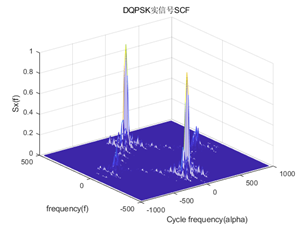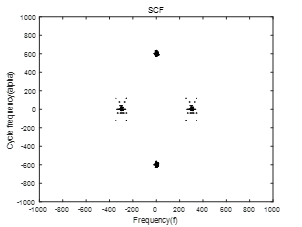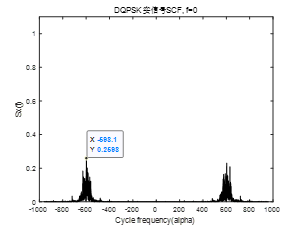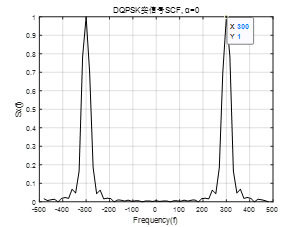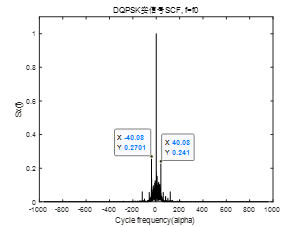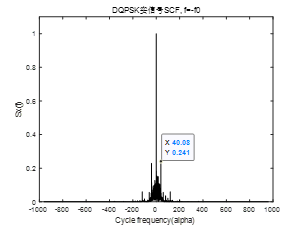符号速率RB = 60，采样率Fs = 960，载波频率fc = 400，符号数N = 1000，矩形成形，二倍载波频率不为符号速率的整数倍。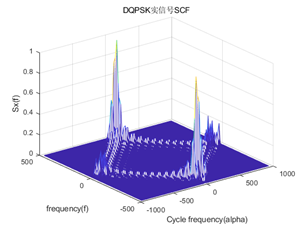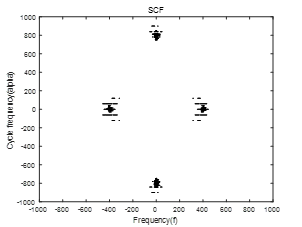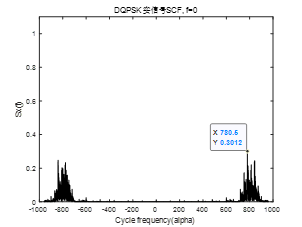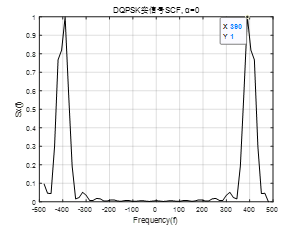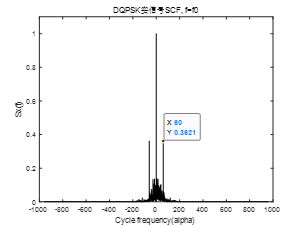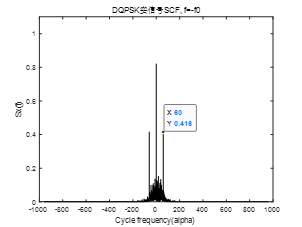符号速率RB = 60，采样率Fs = 960，载波频率fc = 300，符号数N = 1000，滚降系数 ，根升余弦成形，二倍载波频率为符号速率的整数倍。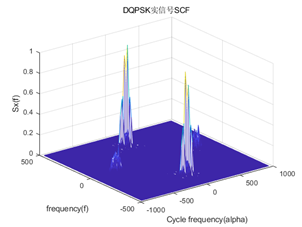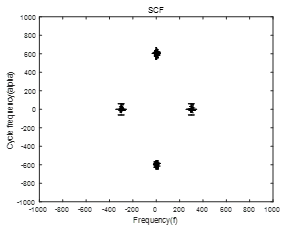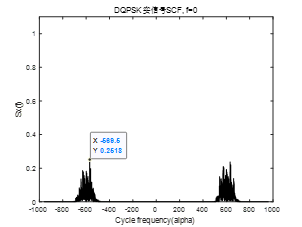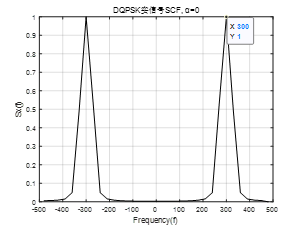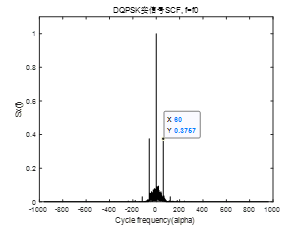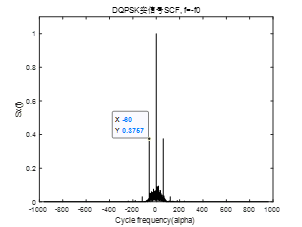8.4.2 复QPSK信号 符号速率RB = 60，采样率Fs = 960，载波频率fc = 400，符号数N = 1000，矩形成形。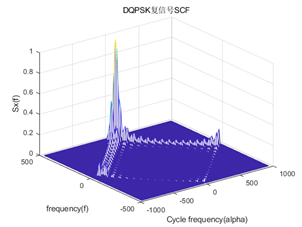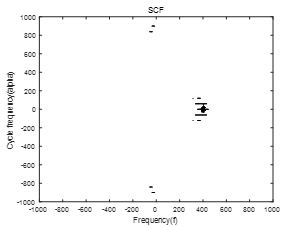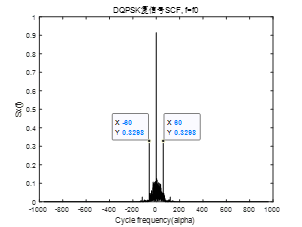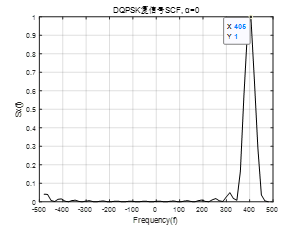符号速率RB = 60，采样率Fs = 960，载波频率fc = 400，符号数N = 1000，根升余弦成形。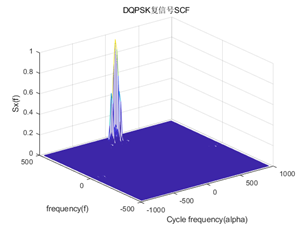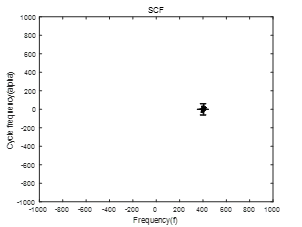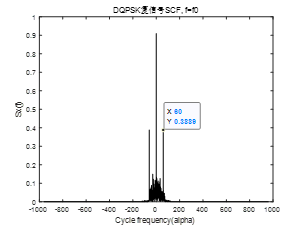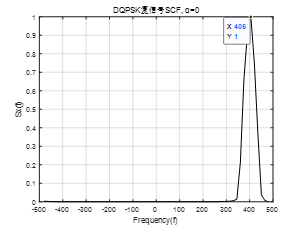8.5 仿真代码 DQPSK实信号 clear;close all;clc; %% 生成dqpsk信号 RB = 60; % 符号速率 beta = 0.8; % 滚降系数 B = (1+beta)*RB; % 带宽 Fs = 960; % 采样率 fc = 300; % 载频 symlen = 1000; % 符号数 rc = randi([0,4-1],1,symlen); fd = Fs/RB; % 过采倍数 Len = symlen*fd; % 总的采样点数 t = 0:1/Fs:(Len-1)/Fs; % 共symlen*fd个样点，1s采样960个点，时间为6.25秒 % 将绝对码变为相对码，采用数组查表的方法 drc = zeros(1,symlen); % 参考比特第1比特设置为1 % 星座映射关系为表7-1，P260 % 绝对码映射到相位变化Δφ 0(1)---0°; 1(2)--- 270°; 2(3)---90°; 3(4)---180° % 如270表示绝对码比特1代表相位变化270°，90表示比特2相位变化90° dphai = [0,270,90,180]; % 当前时刻相位φ映射到星座点 0(1)---0°; 1(2)--- 270°; 2(3)---90°; 3(4)---180° % 如当前时刻相位为180°，则mod(180,360)/90=2，当前相位映射为phaik(2+1)=3 % 式中加1是因为MATLAB坐标从1算起 phaik = [0,2,3,1]; for i = 2:symlen drc(i) = phaik(mod(dphai(rc(i)+1)+dphai(drc(i-1)+1),360)/90+1); end % 将四进制数据分成同相、正交两路数据的双极性码 % I支路在低位，Q支路在高位 drc_i = zeros(1,symlen); drc_q = zeros(1,symlen); for i = 1:symlen drcb = dec2bin(drc(i), 2); drc_i(i) = 1-2*str2double(drcb(2)); drc_q(i) = 1-2*str2double(drcb(1)); end %% 成形滤波 % 矩形成形 for i = 1:symlen sbas_rect_i((i-1)*fd+1:i*fd) = drc_i(i)*ones(1,fd); sbas_rect_q((i-1)*fd+1:i*fd) = drc_q(i)*ones(1,fd); end % 根升余弦成形 h = rcosdesign(beta,5,fd,'sqrt'); up_drc_i = upsample(drc_i,fd); up_drc_q = upsample(drc_q,fd); sbas_rcos_i = filter(h,1,up_drc_i); sbas_rcos_q = filter(h,1,up_drc_q); %% 调制 % 实信号 % dqpsk = sbas_rect_i.*cos(2*pi*fc*t)-sbas_rect_q.*sin(2*pi*fc*t); % 矩形成形 dqpsk = sbas_rcos_i.*cos(2*pi*fc*t)-sbas_rcos_q.*sin(2*pi*fc*t); % 根升余弦成形 figure, plot(-Fs/2:Fs/2-1, fftshift(abs(fft(dqpsk,Fs))),'k'); title('DQPSK实信号频谱'); N = 4096; M = 128; [Sx, alphao, fo] = autofam(dqpsk, Fs, Fs/N*M, Fs/N); % 循环谱 figure; mesh(alphao,fo,abs(Sx)); title('DQPSK实信号SCF');xlabel('Cycle frequency(alpha)'); ylabel('frequency(f)');zlabel('Sx(f)');grid on; figure; contour(fo,alphao,abs(Sx).',[0.1 0.1],'k'); axis([-1000 1000 -1000 1000]);title('SCF'); xlabel('Frequency(f)');ylabel('Cycle frequency(alpha)'); %% f = 0 截面 f0 = (length(fo)+1 )/2; figure; plot(alphao,abs(Sx(f0,:)),'k');title('DQPSK实信号SCF, f=0'); xlabel('Cycle frequency(alpha)');ylabel('Sx(f)');ylim([0 1.1]); %% f = f0 截面 Nf = pow2(nextpow2(N/M)); % f 轴分辨率 1/Np L = Nf/4; P = pow2(nextpow2(4 * N/Nf)); Na = P*L; % α 轴分辨率 1/Na ff0 = floor((fc+Fs/2)*Nf/Fs+1); % -480+Fs/Np*(k-1) = 300 figure; plot(alphao,abs(Sx(ff0,:)),'k');title('DQPSK实信号SCF, f=f0'); xlabel('Cycle frequency(alpha)');ylabel('Sx(f)');ylim([0 1.1]); %% f = -f0 截面 ff0 = floor((-fc+Fs/2)*Nf/Fs+1); % -480+Fs/Np*(k-1) = -300 figure; plot(alphao,abs(Sx(ff0,:)),'k');title('DQPSK实信号SCF, f=-f0'); xlabel('Cycle frequency(alpha)');ylabel('Sx(f)');ylim([0 1.1]); %% alpha = 0 截面 a0 = (length(alphao)+1)/2; figure; plot(fo,abs(Sx(:,a0)),'k');title('DQPSK实信号SCF, α=0'); xlabel('Frequency(f)');ylabel('Sx(f)');grid on;  DQPSK复信号 clear;close all;clc; %% 生成dqpsk信号 RB = 60; % 符号速率 beta = 0.8; % 滚降系数 B = (1+beta)*RB; % 带宽 Fs = 960; % 采样率 fc = 400; % 载频 symlen = 1000; % 符号数 rc = randi([0,4-1],1,symlen); fd = Fs/RB; % 过采倍数 Len = symlen*fd; % 总的采样点数 t = 0:1/Fs:(Len-1)/Fs; % 共symlen*fd个样点，1s采样960个点，时间为6.25秒 % 将绝对码变为相对码，采用数组查表的方法 drc = zeros(1,symlen); % 参考比特第1比特设置为1 % 星座映射关系为表7-1，P260 % 绝对码映射到相位变化Δφ 0(1)---0°; 1(2)--- 270°; 2(3)---90°; 3(4)---180° % 如270表示绝对码比特1代表相位变化270°，90表示比特2相位变化90° dphai = [0,270,90,180]; % 当前时刻相位φ映射到星座点 0(1)---0°; 1(2)--- 270°; 2(3)---90°; 3(4)---180° % 如当前时刻相位为180°，则mod(180,360)/90=2，当前相位映射为phaik(2+1)=3 % 式中加1是因为MATLAB坐标从1算起 phaik = [0,2,3,1]; for i = 2:symlen drc(i) = phaik(mod(dphai(rc(i)+1)+dphai(drc(i-1)+1),360)/90+1); end % 将四进制数据分成同相、正交两路数据的双极性码 % I支路在低位，Q支路在高位 drc_i = zeros(1,symlen); drc_q = zeros(1,symlen); for i = 1:symlen drcb = dec2bin(drc(i), 2); drc_i(i) = 1-2*str2double(drcb(2)); drc_q(i) = 1-2*str2double(drcb(1)); end %% 成形滤波 % 矩形成形 for i = 1:symlen sbas_rect_i((i-1)*fd+1:i*fd) = drc_i(i)*ones(1,fd); sbas_rect_q((i-1)*fd+1:i*fd) = drc_q(i)*ones(1,fd); end % 根升余弦成形 h = rcosdesign(beta,5,fd,'sqrt'); up_drc_i = upsample(drc_i,fd); up_drc_q = upsample(drc_q,fd); sbas_rcos_i = filter(h,1,up_drc_i); sbas_rcos_q = filter(h,1,up_drc_q); %% 调制 % 复信号 % dqpsk_c = (sbas_rect_i+1j*sbas_rect_q).*exp(1j*2*pi*fc*t); % 矩形成形 dqpsk_c = (sbas_rcos_i+1j*sbas_rcos_q).*exp(1j*2*pi*fc*t); % 根升余弦成形 figure, plot(-Fs/2:Fs/2-1, fftshift(abs(fft(dqpsk_c,Fs))),'k'); title('DQPSK复信号频谱'); N = 4096; M = 64; [Sx, alphao, fo] = autofam(dqpsk_c, Fs, Fs/N*M, Fs/N); % 循环谱 figure; mesh(alphao,fo,abs(Sx)); title('DQPSK复信号SCF');xlabel('Cycle frequency(alpha)'); ylabel('frequency(f)');zlabel('Sx(f)');grid on; figure; contour(fo,alphao,abs(Sx).',[0.1 0.1],'k'); axis([-1000 1000 -1000 1000]);title('SCF'); xlabel('Frequency(f)');ylabel('Cycle frequency(alpha)'); %% f = 0 截面 f0 = (length(fo)+1 )/2; figure; plot(alphao,abs(Sx(f0,:)),'k');title('DQPSK复信号SCF, f=0'); xlabel('Cycle frequency(alpha)');ylabel('Sx(f)'); % ylim([0 1.1]); %% f = f0 截面 Nf = pow2(nextpow2(N/M)); % f 轴分辨率 1/Np L = Nf/4; P = pow2(nextpow2(4 * N/Nf)); Na = P*L; % α 轴分辨率 1/Na ff0 = floor((fc+Fs/2)*Nf/Fs+1); % -480+Fs/Np*(k-1) = 300 figure; plot(alphao,abs(Sx(ff0,:)),'k');title('DQPSK复信号SCF, f=f0'); xlabel('Cycle frequency(alpha)');ylabel('Sx(f)'); % ylim([0 1.1]); %% f = -f0 截面 ff0 = floor((-fc+Fs/2)*Nf/Fs+1); % -480+Fs/Np*(k-1) = -300 figure; plot(alphao,abs(Sx(ff0,:)),'k');title('DQPSK复信号SCF, f=-f0'); xlabel('Cycle frequency(alpha)');ylabel('Sx(f)'); % ylim([0 1.1]); %% alpha = 0 截面 a0 = (length(alphao)+1)/2; figure; plot(fo,abs(Sx(:,a0)),'k');title('DQPSK复信号SCF, α=0'); xlabel('Frequency(f)');ylabel('Sx(f)');grid on;  FAM法计算循环谱子函数（未仔细研究推导该算法，计算速度挺快）  function [Sx,alphao,fo] = autofam(x,fs,df,dalpha) %************************************************ % x为输入信号向量 % fs为采样率 % df为频率分辨率 % dalpha为循环频率分辨率 % 注意：需满足df>>dalpha才能得到较高的效果，fs/dalpha为进行采样计算功率谱的码元个数 %%fam算法比ssca(autossca)算法快,但效果一样.都是求循环平稳与谱 %************************************************ % if nargin > 4 | nargin < 4 % error('Wrong number of arguments.'); % end % fs=1280; % fc=160; % N=8192; % PW=N/fs; % signal_type=5; % switch signal_type % case 1; input_signal = sin(2*pi*(fc)*((0:(N-1))/fs)); % case 2; input_signal = bpsk_generator(fs*10^6,fc*10^6,PW*10^3); % case 3; input_signal = qpsk_generator(fs*10^6,fc*10^6,PW*10^3); % case 4; input_signal = lfm_generator(fs) ; % case 5; input_signal = nlfm_generator(fs) ; % otherwise; % end % x = awgn(input_signal,10); % M=128; % dalpha=fs/N; % df=dalpha*M; %-----------Definition of Parameters-----------% Np = pow2(nextpow2(fs/df)); %输入信道数 L = Np/4; %抽取因子，同一行连续列的相邻点的偏移，L表示每次滑动的数据点数 P = pow2(nextpow2(fs/dalpha/L));%信道矩阵的列数,P表示滑动次数 N = P*L; %输入数据的点数 %----------Input Channelization----------------% if length(x) < N x(N) = 0; elseif length(x) > N x = x(1:N); end NN = (P-1)*L+Np; xx = x; xx(NN) = 0; xx = xx(:); X = zeros(Np,P); for k = 0:P-1 X(:,k+1) = xx(k*L+1:k*L+Np); %输入数据xx的1到Np存入X第一列，L+1到L+Np存入第二列，2L+1到2L+Np存入第三列，以此类推。即L为偏移，Np为每段长度。 end %------------------Windowing------------------% % a = hamming(Np); a=kaiser(Np); XW = diag(a)*X; %每段加窗,减少频率泄露 % XW = X; %不加窗，可与加窗的效果作比较 %---------------第一次傅里叶变换--------------------% XF1 = fft(XW); % clear XW; XF1 = fftshift(XF1); XF1 = [XF1(:,P/2+1:P) XF1(:,1:P/2)]; %这两行的功能是把傅里叶变换的结果上半块和下半块交换,左半块和右半块互换 %-------------下变频------------------% E = zeros(Np,P); for k = -Np/2:Np/2-1 for m = 0:P-1 E(k+Np/2+1,m+1) = exp(-i*2*pi*k*m*L/Np); end end XD = XF1.*E; % clear XF1; XD = conj(XD'); %XD转置的复共轭 % clear ('XF1', 'E', 'XW', 'X', 'x'); %-----------乘积运算--------------------% XM = zeros(P,Np^2); for k = 1:Np for l = 1:Np XM(:,(k-1)*Np+l) = (XD(:,k).*conj(XD(:,l))); end end % clear XD; %-----------第二次傅里叶变换-----------------------% XF2 = fft(XM); % clear XM; XF2 = fftshift(XF2); XF2 = [XF2(:,Np^2/2+1:Np^2) XF2(:,1:Np^2/2)]; % length(XF2); XF2 = XF2(P/4:3*P/4,:); % M = abs(XF2); M = XF2; % clear XF2; %Absolute value and complex magnitude %%%%%%%%%%%%%%%%频率分辨率和循环频率分辨率%%%%%%%%%% alphao = (-1:1/N:1)*fs; %about the variable N!!! fo = (-0.5:1/Np:0.5)*fs; Sx = zeros(Np+1,2*N+1); %about the variable N!!! for k1 = 1:P/2+1 for k2 = 1:Np^2 if rem(k2,Np) == 0 l = Np/2-1; else l = rem(k2,Np)-Np/2-1; end k = ceil(k2/Np)-Np/2-1; p = k1-P/4-1; alpha = (k-l)/Np+(p-1)/L/P; f = (k+l)/2/Np; if alpha < -1 || alpha > 1 k2 = k2+1; elseif f < -0.5 || f > 0.5 k2 = k2+1; % elseif rem(k+l,2)==0 && rem(1+N*(alpha+1),1)==0 else kk = 1+Np*(f+0.5); ll = 1+N*(alpha + 1); Sx(round(kk), round(ll)) = M(k1,k2); end end end Sx = Sx./max(max(Sx)); % Normalizes the magnitudes of the values in output matrix (maximum = 1)  参考文献  Gardner W. A . The spectral correlation theory of cyclostationary time-series.  WILLIAM A. GARDNER -Spectral Correlation of Modulated Signals: Part I-Analog Modulation  WILLIAM A. GARDNER -Spectral Correlation of Modulated Signals: Part II-Digital Modulation 文献  给出了深入的介绍性处理，这是充分理解谱相关概念的先决条件。  展开全文QPSK matlab • 7 BPSK信号相关密度函数 7.1 实信号模型 BPSK实信号表达式可以写为 r(t)=y(t)+n(t)r(t) = y(t) + n(t)r(t)=y(t)+n(t) =s(t)p(t)+n(t)= s(t)p(t) + n(t)=s(t)p(t)+n(t) =∑n=−∞∞a(nT)q(t−nT−t0)cos⁡(2πf0t+... 说明：接上一节循环自相关函数和谱相关密度（一）——公式推导 7 BPSK信号谱相关密度函数 7.1 实信号模型 BPSK实信号表达式可以写为 $r(t) = y(t) + n(t)$ $= s(t)p(t) + n(t)$ $= \sum\limits_{n = - \infty }^\infty {a(nT)q(t - nT - {t_0})} \cos (2\pi {f_0}t + \theta ){\text{ + }}n(t)$(22) 其中，${t_0}$为起始时间，$T$为符号速率，$a(n)$为基带符号序列，${f_0}$为载波频率，$\theta$为初始相位，$n(t)$为高斯白噪声，$q(t)$为矩形脉冲，其表达式和傅里叶变换为 $q(t)=\left\{\begin{array}{ll}1, & |t| \leq T / 2 \\ 0, & |t|>T / 2\end{array}\right.$ (23) $Q(f) = T\operatorname{Sa} (\pi fT)$ (24) 且 $s(t) = \sum\limits_{n = - \infty }^\infty {a(nT)q(t - nT - {t_0})}$ $= q(t - {t_0}) \otimes \sum\limits_n {a(t)\delta (t - nT)}$ $= q(t - {t_0}) \otimes \hat a(t)$ (25) $p(t) = \cos (2\pi {f_0}t + \theta )$ (26) 由(21)知，基带脉冲序列$a(nT)$的谱相关密度函数为 $\tilde S_a^\alpha (f) = \frac{1}{T}\sum\limits_{n = - \infty }^\infty {\sum\limits_{m = - \infty }^\infty {S_a^{\alpha {\text{ + }}m/T}(f - \frac{m}{{2T}} - \frac{n}{T})} }$ (27) 由(19)可知，$a(t)$以周期$T$理想抽样后的谱相关密度函数为 $S_{\hat a}^\alpha (f) = \frac{1}{{{T^2}}}\sum\limits_{n = - \infty }^\infty {\sum\limits_{m = - \infty }^\infty {S_{\hat a}^{\alpha {\text{ + }}m/T}(f - \frac{m}{{2T}} - \frac{n}{T})} }$ (28) 根据傅里叶变换的时移性质，$q(t - {t_0})$的傅里叶变换为$Q(f){e^{ - j2\pi f{t_0}}}$，则(5)由可得$s(t)$的谱相关密度函数为 $S_s^\alpha (f) = \frac{1}{T}Q(f + \alpha /2){Q^*}(f - \alpha /2){e^{ - j2\pi \alpha {t_0}}}\tilde S_a^\alpha (f)$ (29) 考虑$p(t)$的二次变换 ${v_\tau }(t) = p(t{\text{ + }}\tau /2){p^*}(t - \tau /2)$ $= \frac{1}{4}({e^{j2\pi {f_0}\tau }} + {e^{ - j2\pi {f_0}\tau }} + {e^{j(4\pi {f_0}t + 2\theta )}} + {e^{ - j(4\pi {f_0}t + 2\theta )}})$ (30) 其Fourier级数系数为 ${\left\langle {{v_\tau }(t){e^{ - j2\pi \alpha t}}} \right\rangle _t} = \frac{1}{4}{e^{j2\pi {f_0}\tau }}{\left\langle {{e^{ - j2\pi \alpha t}}} \right\rangle _t} + \frac{1}{4}{e^{ - j2\pi {f_0}\tau }}{\left\langle {{e^{ - j2\pi \alpha t}}} \right\rangle _t}$ $+ \frac{1}{4}{e^{ - j2\theta }}{\left\langle {{e^{ - j2\pi (\alpha + 2{f_0})t}}} \right\rangle _t} + \frac{1}{4}{e^{j2\theta }}{\left\langle {{e^{ - j2\pi (\alpha - 2{f_0})t}}} \right\rangle _t}$(31) 则$p(t)$的循环自相关函数和谱相关密度函数为 $R_{p}^{\alpha}(\tau)=\left\{\begin{array}{cc}\frac{1}{4} e^{\pm j 2 \theta} & \alpha=\pm 2 f_{0} \\ \frac{1}{2} \cos \left(2 \pi f_{0} \tau\right) & \alpha=0 \\ 0 & \text { otherwise }\end{array}\right.$(32) $S_{p}^{\alpha}(f)=\left\{\begin{array}{cc}\frac{1}{4} e^{\pm j 2 \theta} \delta(f) & \alpha=\pm 2 f_{0} \\ \frac{1}{4}\left[\delta\left(f+f_{0}\right)+\delta\left(f-f_{0}\right)\right] & \alpha=0 \\ 0 & \text { otherwise }\end{array}\right.$(33) 由(12)、(13)得$y(t)$的循环自相关函数为 $R_y^\alpha (\tau ) = \sum\limits_\beta {R_p^\beta (\tau )R_s^{\alpha - \beta }(\tau )}$ $= \frac{1}{4}{e^{j2\theta }}R_s^{\alpha - 2{f_0}}(\tau ) + \frac{1}{4}{e^{ - j2\theta }}R_s^{\alpha + 2{f_0}}(\tau ) + \frac{1}{2}\cos (2\pi {f_0}\tau )R_s^\alpha (\tau )$ (34) $S_y^\alpha (f) = \sum\limits_\beta {S_p^\beta (f) \otimes S_s^{\alpha - \beta }(f)}$ $= \frac{1}{4}\left[ {S_s^\alpha (f + {f_0}) + S_s^\alpha (f - {f_0}) + {e^{j2\theta }}S_s^{\alpha - 2{f_0}}(f) + {e^{ - j2\theta }}S_s^{\alpha + 2{f_0}}(f)} \right]$ (35) 将(29)代入(35)，得$y(t)$的谱相关密度函数为 $S_y^\alpha (f) = \frac{1}{{4T}}\{ [Q(f + {f_0} + \alpha /2){Q^*}(f + {f_0} - \alpha /2)\tilde S_a^\alpha (f + {f_0})$ $Q(f - {f_0} + \alpha /2){Q^*}(f - {f_0} - \alpha /2)\tilde S_a^\alpha (f - {f_0})]{e^{ - j2\pi \alpha {t_0}}}$ $Q(f + {f_0} + \alpha /2){Q^*}(f - {f_0} - \alpha /2)\tilde S_a^{\alpha + 2{f_0}}(f){e^{ - j[2\pi (\alpha + 2{f_0}){t_0} + 2\theta ]}}$ $Q(f - {f_0} + \alpha /2){Q^*}(f + {f_0} - \alpha /2)\tilde S_a^{\alpha - 2{f_0}}(f){e^{ - j[2\pi (\alpha - 2{f_0}){t_0} - 2\theta ]}}\}$ (36) 对于01先验等概的基带符号序列$a(n)$，其循环自相关函数为 $\tilde R_a^\alpha (kT) = \mathop {\lim }\limits_{N \to \infty } \frac{1}{{2N + 1}}\sum\limits_{n = - N}^N {a(nT + kT)a(nT)} {e^{ - j2\pi \alpha (n + k/2)T}}$ (37) 当且仅当$k = 0$且$\alpha = m/T$时，$\tilde R_a^\alpha (kT) = {\tilde R_a}(0)$，则其谱相关密度函数为 \tilde{S}_{a}^{\alpha}(f)=\left\{\begin{aligned} \tilde{R}_{a}(0)=1, & \alpha=m / T \\ 0, & \alpha \neq m / T \end{aligned}\right.(38) 对于高斯白噪声$n(t)$，当且仅当$\alpha = 0$时，其谱相关密度函数不为零，则BPSK实信号的谱相关密度函数为 $S_{r}^{\alpha}(f)=\left\{\begin{array}{cc}S_{y}^{\alpha}(f)+S_{n}^{\alpha}(f), & \alpha=0 \\ S_{y}^{\alpha}(f), & \alpha \neq 0\end{array}\right.$(39) 由此可见，噪声$n(t)$只影响循环频率为零时的截面。 7.2 复信号模型 BPSK复信号表达式可以写为 $r(t) = y(t) + n(t)$ ${\text{ = }}s(t)p(t) + n(t)$ $= \sum\limits_{n = - \infty }^\infty {a(nT)q(t - nT - {t_0})} {e^{j(2\pi {f_0}t + \theta )}}$ (40) 同理，${t_0}$为起始时间，$T$为符号速率，$a(n)$为基带符号序列，${f_0}$为载波频率，$\theta$为初始相位，$n(t)$为高斯白噪声，$q(t)$为矩形脉冲。令 $p(t) = {e^{j(2\pi {f_0}t + \theta )}}$ (41) 同实数信号模型对比，只有$p(t)$发生了改变，其二次变换的其傅里叶级数系数为 ${\left\langle {{v_\tau }(t){e^{ - j2\pi \alpha t}}} \right\rangle _t} = {\left\langle {p(t{\text{ + }}\tau /2){p^*}(t - \tau /2){e^{ - j2\pi \alpha t}}} \right\rangle _t}$ $= {e^{j2\pi {f_0}\tau }}{\left\langle {{e^{ - j2\pi \alpha t}}} \right\rangle _t}$ (42) 则$p(t)$的循环自相关函数和谱相关密度函数为 $R_{p}^{\alpha}(\tau)=\left\{\begin{array}{cc}e^{j 2 \pi f_{0} \tau} & \alpha=0 \\ 0 & \alpha \neq 0\end{array}\right.$(43) $S_{p}^{\alpha}(f)=\left\{\begin{array}{cc}\delta\left(f-f_{0}\right) & \alpha=0 \\ 0 & \alpha \neq 0\end{array}\right.$(44) 由(12)、(13)得$y(t)$的循环自相关函数为 $R_y^\alpha (\tau ) = \sum\limits_\beta {R_p^\beta (\tau )R_s^{\alpha - \beta }(\tau )} = {e^{j2\pi {f_0}\tau }}R_s^\alpha (\tau )$ (45) $S_y^\alpha (f) = \sum\limits_\beta {S_p^\beta (f) \otimes S_s^{\alpha - \beta }(f)}$ $= \delta (f - {f_0}) \otimes S_s^\alpha (f)$ $= S_s^\alpha (f - {f_0})$ (46) 将(29)代入(46)，得$y(t)$的谱相关密度函数为 $S_y^\alpha (f) = \frac{1}{T}[Q(f - {f_0} + \alpha /2){Q^*}(f - {f_0} - \alpha /2){e^{ - j2\pi \alpha {t_0}}}\tilde S_a^\alpha (f - {f_0})]$ (47) 同(39)，可得复BPSK信号的谱相关密度函数为 $S_{r}^{\alpha}(f)=\left\{\begin{array}{cc}S_{y}^{\alpha}(f)+S_{n}^{\alpha}(f), & \alpha=0 \\ S_{y}^{\alpha}(f), & \alpha \neq 0\end{array}\right.$(48) 7.3 谱分析 7.3.1 主峰个数 对于实BPSK信号，由(36)、(38)可知，其谱相关密度函数在$f = 0$且$\alpha = \pm 2{f_0}$处各有一个主峰；在$\alpha = 0$且$f = \pm {f_0}$处各有一个主峰，即实BPSK信号共有4个主峰。 对于复BPSK信号，由(47)、(48)可知，其谱相关密度函数只有在$f = {f_0}$且$\alpha = 0$处有一个谱峰。 7.3.2 切面特征 在式(36)中，令$f = 0$，$\alpha = \pm 2{f_0} + m/T$得 $S_{y}^{\alpha}(f)=\left\{\begin{array}{ll}\frac{1}{4 T}\left|Q\left(-f_{0}+\alpha / 2\right)\right|^{2} e^{-j\left[2 \pi n t_{0} / T-2 \theta\right]} & \alpha=2 f_{0}+m / T \\ \frac{1}{4 T}\left|Q\left(f_{0}+\alpha / 2\right)\right|^{2} e^{-j\left[2 \pi n t_{0} / T+2 \theta\right]} & \alpha=-2 f_{0}+m / T\end{array}\right.$(49) 特别地，当$m = 0$时，有 $S_{y}^{\alpha}(f)=\left\{\begin{array}{ll}\frac{1}{4 T}|Q(0)|^{2} e^{j 2 \theta} & \alpha=2 f_{0} \\ \frac{1}{4 T}|Q(0)|^{2} e^{-j 2 \theta} & \alpha=-2 f_{0}\end{array}\right.$(50) 即在$f = 0$切面，其谱相关密度函数幅度最大值出现在循环频率为$\alpha = \pm 2{f_0}$处，由此可估计实BPSK信号的载波频率；在其左右偏移符号速率处，出现次峰值，可估计其符号速率，且可根据$\alpha = \pm 2{f_0}$处对应的谱相关密度函数的相位来估计初相$\theta$。 令$f = \pm {f_0}$，$\alpha = m/T$得 $S_y^\alpha (f) = \frac{1}{{4T}}\{ [Q(2{f_0} + \alpha /2){Q^*}(2{f_0} - \alpha /2) + |Q(\alpha /2){|^2}]{e^{ - j2\pi \alpha {t_0}}}$ (51) 即在$f = \pm {f_0}$切面，其谱相关密度函数幅度在循环频率为$\alpha = m/T$即符号速率整数倍处出现峰值，在$\alpha = 0$处的峰值最大，由此可估计实BPSK信号的符号速率，此外还可根据符号速率处对应的谱相关密度函数的相位来估计时延${t_0}$；其中，需要注意的是，当频率分辨率远远小于循环频率分辨率，即$\Delta f \gg \Delta \alpha$时，符号速率处对应的峰值才比较明显。 对于复BPSK信号，在式(47)中，令$\alpha = 0$，得 $S_y^\alpha (f) = \frac{1}{T}|Q(f - {f_0}){{\text{|}}^2}$ (52) 即在$\alpha = 0$切面，其谱相关密度函数幅度只在$f = {f_0}$出现峰值，由此可估计复BPSK信号的载波频率，但此时噪声$n(t)$的谱相关密度函数不为零，因此利用该切面进行载频估计受噪声影响较大。 令$f = {f_0}$，得 $S_y^\alpha (f) = \frac{1}{T}|Q(\alpha /2){|^2}{e^{ - j2\pi \alpha {t_0}}}\tilde S_a^\alpha (0)$ (53) 即在$f = {f_0}$切面，其谱相关密度函数幅度在循环频率为$\alpha = m/T$即符号速率整数倍处出现峰值，在$\alpha = 0$处的峰值最大，由此可估计实BPSK信号的符号速率，此外还可根据符号速率处对应的谱相关密度函数的相位来估计时延${t_0}$。 7.4 成形滤波器对谱相关密度函数的影响 无论是BPSK还是QPSK调制信号，对于矩形成形，其频谱为Sa函数，当$\left| f \right| > 1/T$时，存在衰减较慢的旁瓣，因此在循环频率为$\alpha = m/T$或$\alpha = m/T \pm 2{f_0}$处其谱相关密度函数仍然不为零，即在主峰周围会有很多小峰。对于（根）升余弦成形，当$\left| f \right| > 1/T$时，其频谱较快衰减为零，因此其谱相关密度函数只在循环频率为$\alpha = 1/T$或$\alpha = 1/T \pm 2{f_0}$处有值。  展开全文bpsk • z^\alpha (f) = H(f + \alpha /2){H^*}(f - \alpha /2)S_x^\alpha (f)Szα​(f)=H(f+α/2)H∗(f−α/2)Sxα​(f)(5) 2 周期抽样函数的循环自相关函数和相关密度函数 周期抽样函数可以表示为g(t) = \sum\limits_{...公式推导
• FAM法计算循环谱子函数（未仔细研究推导该算法，计算速度挺快） function [Sx,alphao,fo] = autofam(x,fs,df,dalpha) %************************************************ % x为输入信号向量 % fs为采样率 % df为...bpsk matlab
• 以无线光通信中的副载波调制技术为背景，研究了光强调制正交相移键控信号的循环自相关和循环谱密度函数，并对实际测得的信号的循环谱循环自相关函数进行了分析。结果表明，无线光副载波信号经过大气传输，同时受到...
• # python 服从正太分布下概率密度函数 利用input()函数输入均值和标准差, 多次绘制概率密度函数图形并将图像曲线放置在同一张图中 代码块: """ 绘制正太分布函数曲线图 """ import matplotlib.pyplot as plt import...python 正太分布
• 高斯分布： $f(x) = \frac{1}{\sqrt{2\pi }\sigma }exp(-\frac{(x-\mu)^{2}}{2\sigma^{2}})$ ...一个高斯分布只需线性变换即可化为标准高斯分布，所以只需推导标准高斯分布概率密度的积分。由...
• 在本篇博客中，我将与众位看官分享我自己写的关于用核密度函数加权的直方图的计算 欢饮批评指正！！！ 核密度函数加权直方图在基于Mean shift的跟踪算法中经常用到。请客官查看我的博客： 样本均值漂移的...opencv calcHist
• 之前不懂系统辨识的理论，输入信号随便用了一个阶跃信号，后来发现...下面介绍M序列的matlab产生方法，看到很多论坛产生M序列的程序复用性不高，而matlab就提供了产生M序列的专门函数，这里尝试一下。idinput函数产生系matlab
• 聚类算法概述，K-Means算法，聚类算法的衡量指标，层次聚类方法，密度聚类方法及聚类方法详述。
• 目前的研究涉及到了自相关函数和功率之间的关系，自己不懂，就在网上搜，发现一个作者写的很好，这里转载一下，谢谢这位博主。 http://skpsun.blog.163.com/blog/static/2760055201122043815725/
• ## Matlab常用函数

千次阅读 2014-06-03 10:15:00
Matlab有没有求矩阵行数/列数/维数的函数？ ndims(A)返回A的维数 size(A)返回A各个维的最大元素个数 length(A)返回max(size(A)) [m,n]=size(A)如果A是二维数组，返回行数和列数 nnz(A)返回A中非0元素的个数 ...
• 激活函数神经网络神经元中，输入的 inputs 通过加权，求和后，还被作用了一个函数，这个函数就是激活函数 Activation Function。 为什么要用激活函数神经网络中激活函数的主要作用是提供网络的非线性建模能力，如不...深度学习 神经网络
• ## 核函数

千次阅读 2015-10-15 16:28:12
转自：... ...核函数  (2010-12-23 23:08:30) 标签：  校园 分类： 工作篇 高斯核函数 所谓径向基函数 (Radial Basis Function
• 一. 应用背景 在安防大背景下，对敏感区域人流量的管控是一个重要的课题，防止人群骚乱、踩踏现象的发生，对非预期的人员汇聚进行预警... 本节主要讲基于深度学习的回归方法来实现人群密度检测。二. 人群密度之 CrCrowd Net RNN LSTM ConvLSTM
• ## matlab函数总结

千次阅读 2018-07-28 10:38:58
ndims(A)返回A的维数 size(A)返回A各个维的最大元素个数 length(A)返回max(size(A)) [m,n]=size(A)如果A是二维数组，返回行数和列数 nnz(A)返回A中非0元素的...MATLAB的取整函数:fix(x), floor(x) :,ceil(x) , ro...matlab
• ## MATLAB函数速查手册

千次阅读 多人点赞 2018-03-25 09:06:26matlab
• ## matlab函数

千次阅读 2012-05-19 11:43:43
1.feedback是matlab里专门用来求线性时不变系统的前向传递函数的，建立系统的闭环传递函数，不能用来做变名，不能赋值 2.tf（）建立开环传递函数 A a abs 绝对值, 模 acos 反余弦 acosh 反双曲余弦 acot 反余...matlab pascal branch
• ## OPNET核心函数

千次阅读 2014-06-29 19:36:20
OPNET Modeler 核心函数 1. 核心函数简介 1.1 命名规则 OPNET 中的核心函数具有非常标准的命名规则，以增强函数在 C/C++代码中的可视性， 避免名称与非 OPNET 函或变量冲突。以下列出了一些简单的命名规则： ? ? ...
• 通过glutMainLoop下一次循环时，窗口显示将被回调以重新显示窗口的正常面板。多次调用glutPostRedisplay，在下一个显示回调只产生单一的重新显示回调。void glFlush( void );glFlush是OpenGL中的函数，用于强制刷新...
• 大家知道算法的书籍大多是通过C或C++来提供源码的，贪心算法经常会涉及到多个计算结果的对比然后取最优解，C++的处理方式一般都是函数指针，提供一个类似函数指针的数组，然后遍历循环每个函数获得result的结果集。...java 贪心算法 接口
• 包括各种函数、命令含义、还有simulink模块的，希望有帮助 A a abs 绝对值, 模 acos 反余弦 acosh 反双曲余弦 acot 反余切 acoth 反双曲余切 acsc 反余割 acsch 反...matlab pascal branch
• ## R语言中的常用函数

千次阅读 2017-03-22 13:24:42
语言的数学运算和一些简单的函数整理如下： 向量可以进行那些常规的算术运算，不同长度的向量可以相加，这种情况下最短的向量将被循环使用。   改变编译环境的语言（英语） Sys.setenv(LANGUAGE="en") ......